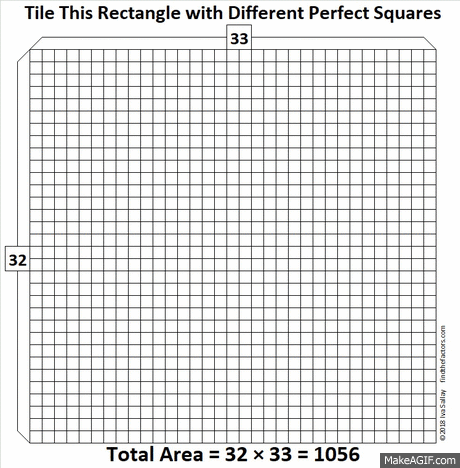# 1056 How to Tile a 32 × 33 Floor

32 × 33 = 1056, and OEIS.org informs us that those are the smallest rectangular dimensions that can be tiled with different perfect squares.

It isn’t difficult to do the tiling. All you have to remember is 32 × 33 and to put an 18 × 18 tile in a corner. The rest of the perfect square tiles seem to almost fall into place as this gif I made illustrates:make science GIFs like this at MakeaGif
Now I’ll tell you a little about the number 1056:
• 1056 is a composite number.
• Prime factorization: 1056 = 2 × 2 × 2 × 2 × 2 × 3 × 11, which can be written 1056 = 2⁵ × 3 × 11
• The exponents in the prime factorization are 5, 1, and 1. Adding one to each and multiplying we get (5 + 1)(1 + 1)(1 + 1) = 6 × 2 × 2 = 24. Therefore 1056 has exactly 24 factors.
• Factors of 1056: 1, 2, 3, 4, 6, 8, 11, 12, 16, 22, 24, 32, 33, 44, 48, 66, 88, 96, 132, 176, 264, 352, 528, 1056
• Factor pairs: 1056 = 1 × 1056, 2 × 528, 3 × 352, 4 × 264, 6 × 176, 8 × 132, 11 × 96, 12 × 88, 16 × 66, 22 × 48, 24 × 44, or 32 × 33
• Taking the factor pair with the largest square number factor, we get √1056 = (√16)(√66) = 4√66 ≈ 32.49615
Since 1056 is the product of consecutive numbers, 32 × 33, it is the sum of the first 32 even numbers:
2 + 4 + 6 + 8 + 10 + . . .  +56 + 58 + 60 + 62 + 64 = 1056

This site uses Akismet to reduce spam. Learn how your comment data is processed.